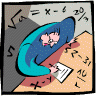## Mathematics, Department of## Dissertations, Theses, and Student Research Papers in Mathematics

8-2012

#### Comments

A DISSERTATION Presented to the Faculty of The Graduate College at the University of Nebraska In Partial Fulfillment of Requirements For the Degree of Doctor of Philosophy, Major: Mathematics, Under the Supervision of Professors Sylvia Wiegand and Mark Walker: Lincoln, Nebraska: August, 2012

Copyright (c) 2012 Ela Celikbas

#### Abstract

This thesis concerns three topics in commutative algebra:

1) The projective line over the integers (Chapter 2),

2) Prime ideals in two-dimensional quotients of mixed power series-polynomial rings (Chapter 3),

3) Fiber products and connected sums of local rings (Chapter 4),

In the first chapter we introduce basic terminology used in this thesis for all three topics.

In the second chapter we consider the partially ordered set (poset) of prime ideals of the projective line Proj(Z[h,k]) over the integers Z, and we interpret this poset as Spec(Z[x]) U Spec(Z[1/x]) with an appropriate identification.

We have some new results that support Aihua Li and Sylvia Wiegand's conjecture regarding the characterization of Proj(Z[h,k]). In particular we show that a possible axiom for Proj(Z[h,k]) proposed by Arnavut, Li and Wiegand holds for some previously unknown cases.

We study the sets of prime ideals of polynomial rings, power series rings and mixed power series-polynomial rings in Chapter 3. Let R be a one-dimensional Noetherian domain and let x and y be indeterminates. We describe the prime spectra of certain two-dimensional quotients of mixed power series/polynomial rings over R, that is, Spec(R[[x]][y]/Q) and Spec(R[y][[x]]/Q'), where Q and Q' are certain height-one prime ideals of R[[x]][y] and R[y][[x]] respectively.

In the last chapter we describe some ring-theoretic and homological properties of fiber products and connected sums of local rings. For Gorenstein Artin k-algebras R and S where k is a field, the connected sum, R#k S, is a quotient of the classical fiber product RXk S. We give basic properties of connected sums over a field and show that certain Gorenstein local k-algebras decompose as connected sums. We generalize structure theorems given by Sally, Elias and Rossi that show two types of Gorenstein local k-algebras are connected sums.

Advisers: Sylvia Wiegand and Mark Walker

COinS